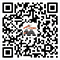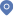Quantum simulation of Maxwell's equations via Schr\"odingerisation首页 - 学术活动

Chuwen Ma, Doctor, Shanghai Jiao Tong University

Weiying Zheng, Professor

Quantum simulation of Maxwell's equations via Schr\"odingerisation

15:00-16:00 September 28 (Thursday), Lecture hall on the first floor of Siyuan Building

We present  quantum algorithms for electromagnetic fields governed by Maxwell's equations. The algorithms are based on the Schr\"odingerisation approach, which transforms any linear PDEs and ODEs with non-unitary dynamics into a system evolving under unitary dynamics, via a warped phase transformation that maps the equation into one higher dimension. In this paper, our quantum algorithms are based on either a direct approximation of Maxwell's equations combined with Yee's algorithm, or a matrix representation in terms of Riemann-Silberstein vectors combined with a spectral approach and an upwind scheme. We implement these algorithms with physical boundary conditions, including perfect conductor and impedance boundaries. We also solve Maxwell's equations for a linear inhomogeneous medium, specifically the interface problem.Several numerical experiments are performed to demonstrate the validity of this approach. In addition, instead of qubits, the quantum algorithms can also be formulated in the continuous variable quantum framework, which allows the quantum simulation of Maxwell's equations in analog quantum simulation.